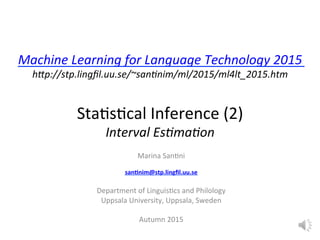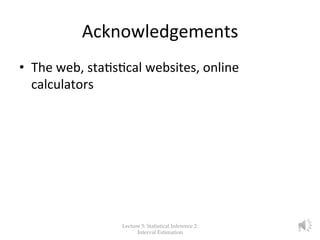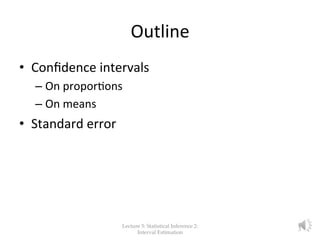Successfully reported this slideshow.

# Lecture 5: Interval Estimation×

# Lecture 5: Interval Estimation

inferential statistics, statistical inference, language technology, interval estimation, confidence interval, standard error, confidence level, z critical value, confidence interval for proportion, confidence interval for the mean, multiplier,

inferential statistics, statistical inference, language technology, interval estimation, confidence interval, standard error, confidence level, z critical value, confidence interval for proportion, confidence interval for the mean, multiplier,

### Lecture 5: Interval Estimation

1. 1. Machine  Learning  for  Language  Technology  2015   h6p://stp.lingﬁl.uu.se/~san?nim/ml/2015/ml4lt_2015.htm       Sta%s%cal  Inference  (2)   Interval  Es?ma?on   Marina  San%ni     san%nim@stp.lingﬁl.uu.se     Department  of  Linguis%cs  and  Philology   Uppsala  University,  Uppsala,  Sweden     Autumn  2015
2. 2. Acknowledgements   •  The  web,  sta%s%cal  websites,  online   calculators     Lecture  5:  Statistical  Inference  2:   Interval  Estimation 2
3. 3. Outline   •  Conﬁdence  intervals   – On  propor%ons   – On  means   •  Standard  error   Lecture  5:  Statistical  Inference  2:   Interval  Estimation 3
4. 4. Sta%s%cal  Inference:     Interval  Es%ma%on   •  Suppose  we  measure  the  error  of  a  classiﬁer  on  a  test   set  and  obtain  a  certain  numerical  error  rate,  eg.  25%.     •  This  corresponds  to  a  success  rate  of  75%.     •  This  is  an  es%mate  on  a  sample  (our  dataset).     •  What  can  we  say  about  the  "true"  success  rate  on  the   target  popula%on?     •  Remember:  We  have  observed  the  propor%on  of   correct  classiﬁca%ons  on  a  sample,  while  the   popula%on  is  unknown  to  us.   Lecture  5:  Statistical  Inference  2:   Interval  Estimation 4
5. 5. Our  prac%cal  ques%on  is…   l  When the estimated success rate is 75%, how close is this value to the true success rate, ie the success rate on the population? ♦  Depends on the amount of sample size Lecture  5:  Statistical  Inference  2:   Interval  Estimation 5
6. 6. What  is  a  conﬁdence  interval?    •  In  sta%s%cal  inference,  one  wishes  to  es%mate  popula%on   parameters  using  observed  sample  data   •  Conﬁdence  intervals  provide  an  essen%al  understanding  of  how   much  faith  we  can  have  in  our  sample  es%mates   •  A  conﬁdence  interval  is  a  range  computed  using  sample  sta%s%cs   to  es%mate  an  unknown  popula%on  parameter  with  a  given  level   of  conﬁdence.     –  For  example,  we  want  to  say:  “we  are  80%  certain  that  true   popula%on  propor%on  falls  within  the  range  of  73.25%  and  76.75%   –  We  usually  write  the  conﬁdence  interval  in  this  way:  [0.732,0.767]         Lecture  5:  Statistical  Inference  2:   Interval  Estimation 6
7. 7. Generally  speaking...   •  A  conﬁdence  interval  is  constructed  by  taking   the  point  es%mate  (p̂)  plus  and  minus  the   margin  of  error.     •  The  margin  of  error  is  computed  by   mul%plying  a  z  mul%plier  by  the   standard  error,  SE(p̂).     Lecture  5:  Statistical  Inference  2:   Interval  Estimation 7
8. 8. Deﬁni%on:  Standard  Error           •  Standard  error  is  a  sta%s%cal  term  that  measures  the   accuracy  with  which  a  sample  represents  a  popula%on.     •  In  sta%s%cs,  a  sample  mean  or  a  sample  propor%on   deviates  from  the  actual  mean  or  propor%on  of  a   popula%on;  this  devia%on  is  the  standard  error.     The  smaller  the  standard  error,  the  more   representa%ve  the  sample  will  be  of  the  overall   popula%on.  The  standard  error  is  also  inversely   propor%onal  to  the  sample  size;  the  larger  the  sample   size,  the  smaller  the  standard  error  because  the   sta%s%c  will  approach  the  actual  value.     Lecture  5:  Statistical  Inference  2:   Interval  Estimation 8
9. 9. The  Mul%plier   The multiplier is a constant that indicates the number of standard deviations in a normal curve. The larger the multiplier, the higher the confidence level, the narrower the confidence interval, the more reliable the prediction of the performace.The constant for 80% percent confidence intervals is 1.28 (see table or use a calculator: http://www.gngroup.com/stat.html ) Lecture  5:  Statistical  Inference  2:   Interval  Estimation 9
10. 10. Conﬁdence  intervals   •  Conﬁdence  intervals  of  a  propor%on   •  Conﬁdence  intervals  of  the  mean   Lecture  5:  Statistical  Inference  2:   Interval  Estimation 10
11. 11. Conﬁdence  interval  for  propor%on   •  A  conﬁdence  interval  for  a  propor%on  is   constructed  by  taking  the  point  es%mate  (p̂)   plus  and  minus  the  margin  of  error.  The   margin  of  error  is  computed  by  mul%plying  a   mul%plier  by  the  standard  error,  SE(pˆ).   Lecture  5:  Statistical  Inference  2:   Interval  Estimation 11
12. 12. The  standard  error  of  propor%on:   p̂  (p-­‐hat)   •  The  standard  error  is  an  es%mate  of  the  standard  devia%on   of  a  sta%s%c.     •  This  is  the  formula  of  the  Standard  Error  of  an  es%mated   propor%on  (the  hat  always  represents  an  es%mate)   •  p̂  =  es%mated  propor%on   •  n  =  sample  (number  of  observa%ons)   Lecture  5:  Statistical  Inference  2:   Interval  Estimation 12
13. 13. Our  prac%cal  ques%on  is…   l  When the estimated success rate is 75%, how close is this value to the true success rate, ie the success rate on the population? ♦  Depends on the amount of sample size Lecture  5:  Statistical  Inference  2:   Interval  Estimation 13
14. 14. Conﬁdence  intervals  on  our   propor%on   l  We can say that our point estimate 75% lies within a certain specified interval with a certain specified confidence (say 80%): l  Example: S=750 successes in N=1000 trials l  Estimated success rate: 75% l  How close is this to true success rate p? l  Answer: with 80% confidence p in [73.2,76.7] l  Another example: S=75 and N=100 l  Estimated success rate: 75% l  Answer: With 80% confidence p in [69.1,80.1] Lecture  5:  Statistical  Inference  2:   Interval  Estimation 14
15. 15. l  p̂ = 75%, n = 1000, confidence = 80% (so that z = 1.28): p∈[0.732,0.767] l  p̂ = 75%, n = 100, confidence = 80% (so that z = 1.28): p∈[0.691,0.801] l  Usually the normal distribution assumption is only valid for large n (i.e. n > 100) l  In a case like this: p̂ = 75%, n = 10, confidence = 80% (so that z = 1.28): p∈[0.549,0.881] Lecture  5:  Statistical  Inference  2:   Interval  Estimation 15
16. 16. Conﬁdence  Interval  Calculator  for  Propor%ons   hdps://www.mccallum-­‐layton.co.uk/tools/sta%s%c-­‐calculators/conﬁdence-­‐interval-­‐for-­‐propor%ons-­‐ calculator/     Lecture  5:  Statistical  Inference  2:   Interval  Estimation 16
17. 17. Conﬁdence  intervals  around  the  mean   Conﬁdence  intervals  are  calculated  based  on  the   standard  error  of  the  mean  (SEM):     s  =  sample  standard  devia%on  (see  formula  below)     n  =  sample  (number  of  observa%ons)     The  following  is  the  sample  standard  devia%on  formula  (see  also  lecture  2):   Lecture  5:  Statistical  Inference  2:   Interval  Estimation 17
18. 18. Example:  How  to  compute  the   conﬁdence  interval  of  teh  mean     A  brand  ra%ng  on  a  ﬁve  point  scale  from  62  par%cipants  was  4.32  with  a  standard  devia%on  of  .845.   What  is  the  95%  conﬁdence  interval?     1)  Find  the  mean:  4.32   2)  Compute  the  standard  devia%on:  .845   3)  Compute  the  standard  error  by  dividing  the  standard  devia%on  by  the  square  root  of  the  sample  size:     .845/  √(62)  =  .11   4)  Compute  the  margin  of  error  by  mul%plying  the  standard  error  by  2  (it  is  common  to  round  up  1.96   to  2).  =  .11  x  2  =  .22   5)  Compute  the  conﬁdence  interval  by  adding  the  margin  of  error  to  the  mean  from  Step  1  and  then   subtrac%ng  the  margin  of  error  from  the  mean:          Lower  limit:  4.32-­‐.22  =  4.10    Upper  limit:  4.32+.22  =  4.54       The  95%  conﬁdence  interval  is  4.10  to  4.54.  We  don't  have  any  historical  data  using  this  5-­‐point   branding  scale,  however,  historically,  scores  above  80%  of  the  maximum  value  tend  to  be  above   average  (4  out  of  5  on  a  5  point  scale).    Therefore  we  can  be  fairly  conﬁdent  that  the  brand  is  at  least   above  the  average  threshold  of  4  because  the  lower  end  of  the  conﬁdence  interval  exceeds  4.     Source:  hdp://www.measuringu.com/blog/ci-­‐ﬁve-­‐steps.php     Lecture  5:  Statistical  Inference  2:   Interval  Estimation 18
19. 19. Conﬁdence  Interval  Calculator  for  Means     hdps://www.mccallum-­‐layton.co.uk/tools/sta%s%c-­‐calculators/conﬁdence-­‐interval-­‐for-­‐mean-­‐calculator/         Lecture  5:  Statistical  Inference  2:   Interval  Estimation 19
20. 20. Quiz  1:  Conﬁdence  Interval  (Mean)   You  take  a  sample  of  25  test  scores  from  a   popula%on.  The  sample  mean  is  38  and  the   populaton  standard  devia%on  is  6.5.  What  is  the   95%  conﬁdence  interval  of  the  mean?     1.  [37.49,38.51]   2.  [36.49,39.51]   3.  [35.45,40.55]   Lecture  5:  Statistical  Inference  2:   Interval  Estimation 20
21. 21. Calculator     hdps://www.mccallum-­‐layton.co.uk/tools/sta%s%c-­‐calculators/conﬁdence-­‐ interval-­‐for-­‐mean-­‐calculator   Lecture  5:  Statistical  Inference  2:   Interval  Estimation 21
22. 22. Quiz  2:  Conﬁdence  Interval   (Propor%on)   747  out  of  1168  female  students  said  they   always  use  a  seatbelt  when  driving.  What  is  the   99%  conﬁdence  interval  for  the  propor%on  of   female  students  in  the  popula%on  who  always   use  a  seatbelt  when  driving?   1.  [.612,.668]   2.  [.604,.676]   3.  None  of  the  above     Lecture  5:  Statistical  Inference  2:   Interval  Estimation 22
23. 23. Calculator   hdps://www.mccallum-­‐layton.co.uk/tools/sta%s%c-­‐calculators/conﬁdence-­‐ interval-­‐for-­‐propor%ons-­‐calculator/       Lecture  5:  Statistical  Inference  2:   Interval  Estimation 23
24. 24. Conclusions   •  A  conﬁdence  interval  is  a  range  of  values  that  is  likely  to  contain  an   unknown  popula%on  parameter.     •  Conﬁdence  intervals  serve  as  good  es%mates  of  the  popula%on   parameter  because  the  procedure  tends  to  produce  intervals  that   contain  the  parameter.     •  Conﬁdence  intervals  are  comprised  of  the  point  es%mate  (the  most   likely  value)  and  a  margin  of  error  around  that  point  es%mate.  The   margin  of  error  indicates  the  amount  of  uncertainty  that  surrounds   the  sample  es%mate  of  the  popula%on  parameter.     We  will  resume  this  topic  in  Lecture  8.   Lecture  5:  Statistical  Inference  2:   Interval  Estimation 24
25. 25. The  end   Lecture  5:  Statistical  Inference  2:   Interval  Estimation 25